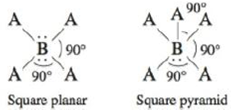# Two variations of the octahedral geometry (see Table 4-1) are illustrated below. Which of the compounds/ions Br 3 − , ClF 3 , XeF 4 , SF 4 , PF 5 , ClF 5 , and SF 6 have these molecular structures?### Chemistry: An Atoms First Approach

2nd Edition
Steven S. Zumdahl + 1 other
Publisher: Cengage Learning
ISBN: 9781305079243

#### Solutions

Chapter
Section### Chemistry: An Atoms First Approach

2nd Edition
Steven S. Zumdahl + 1 other
Publisher: Cengage Learning
ISBN: 9781305079243
Chapter 4, Problem 26E
Textbook Problem
56 views

## Two variations of the octahedral geometry (see Table 4-1) are illustrated below.Which of the compounds/ions Br3−, ClF3, XeF4, SF4, PF5, ClF5, and SF6 have these molecular structures?

Interpretation Introduction

Interpretation: Interpretation: The molecular structure of given compound is to be predicted from given structures.

Concept introduction: Molecular structure is a three-dimensional shape of a molecule.

Bond angle is the angle between atoms of molecule.

To determine: The correct molecular structure of Br3,ClF3,XeF4,SF4,PF5,ClF5 and SF4 (including bond angles) according to given structure.

### Explanation of Solution

The steps are to be followed to determine the molecular structure is:

• Identify the central atom.
• Count its valence electrons.
• Add or subtract electrons for charge.
• Draw the Lewis structure.
• Divide the total number of electrons by 2 to find the number of electron pairs.
• Use this number to predict the shape.

The atomic number of bromine (Br) is 35 and its electronic configuration is,

1s22s22p63s23p63d104s24p5

The valence electron of bromine is 7

The total number of valence electrons is,

3Br+1e=7×3+1=22

The Lewis structure of Br3 is

Figure 1

The formula of number of electron pairs is

X=V+M±C2

Where,

• X is number of electron pairs
• V is valence electrons of central atom
• M is number of monovalent atoms
• C is charge on compound

The number of electron pairs is

X=V+M±C2X=7+12=4

Refer to table (4-1)

The shape of compound is linear and bond angle is 1800

Explanation:

The steps are to be followed to determine the molecular structure is:

• Identify the central atom.
• Count its valence electrons.
• Add or subtract electrons for charge.
• Draw the Lewis structure.
• Divide the total number of electrons by 2 to find the number of electron pairs.
• Use this number to predict the shape.

The atomic number of (central atom) chlorine (Cl) is 17 and its electronic configuration is,

1s22s22p63s23p5

The valence electron of chlorine is 7

The atomic number of fluorine (F) is 9 and its electronic configuration is,

1s22s22p5

The valence electron of fluorine is 7

The total number of valence electrons is,

3Cl+F=7×3+7=28

The Lewis structure of ClF3 is

Figure 2

The formula of number of electron pairs is

X=V+M±C2

Where,

• X is number of electron pairs
• V is valence electrons of central atom
• M is number of monovalent atoms
• C is charge on compound

The number of electron pairs is

X=V+M±C2X=7+32=5

Refer to table (4-1)

The shape of compound is T-shape.

Explanation:

The steps are to be followed to determine the molecular structure is:

• Identify the central atom.
• Count its valence electrons.
• Add or subtract electrons for charge.
• Draw the Lewis structure.
• Divide the total number of electrons by 2 to find the number of electron pairs.
• Use this number to predict the shape.

The atomic number of central atom sulfur (S) is 16 and its electronic configuration is,

1s22s22p63s23p4

The valence electron of sulfur is 6

The atomic number of fluorine (F) is 9 and its electronic configuration is,

1s22s22p5

The valence electron of fluorine is 7

The total number of valence electrons is,

S+4F=6+4×7=34

The Lewis structure of SF4 is

Figure 3

The formula of number of electron pairs is

X=V+M±C2

Where,

• X is number of electron pairs
• V is valence electrons of central atom
• M is number of monovalent atoms
• C is charge on compound

The number of electron pairs is

X=V+M±C2X=6+42=5

Refer to table (4-1)

The shape of compound is see-saw-shape.

Explanation:

The steps are to be followed to determine the molecular structure is:

• Identify the central atom.
• Count its valence electrons.
• Add or subtract electrons for charge.
• Draw the Lewis structure.
• Divide the total number of electrons by 2 to find the number of electron pairs.
• Use this number to predict the shape.

The central atom in XeF4 is xenon (Xe) . The atomic number of xenon is 54 and its electronic configuration is,

1s22s22p63s23p63d104s24p64d105s25p6

The valence electron of xenon is 8

The atomic number of fluorine (F) is 9 and its electronic configuration is,

1s22s22p5

The valence electron of fluorine is 7

The total number of valence electrons is,

Xe+4F=8+4×7=36

The Lewis structure of XeF4 is

### Still sussing out bartleby?

Check out a sample textbook solution.

See a sample solution

#### The Solution to Your Study Problems

Bartleby provides explanations to thousands of textbook problems written by our experts, many with advanced degrees!

Get Started

Find more solutions based on key concepts
Insufficient dietary protein can cause slowed protein synthesis. hepatitis. accelerated growth in children. all...

Nutrition: Concepts and Controversies - Standalone book (MindTap Course List)

What is the difference between a supernova explosion and a nova explosion?

Horizons: Exploring the Universe (MindTap Course List)

A violin string has a length of 0.350 m and is tuned to concert G, with fG = 392 Hz. (a) How far from the end o...

Physics for Scientists and Engineers, Technology Update (No access codes included)

How are neutron stars and white dwarfs similar? How do they differ?

Foundations of Astronomy (MindTap Course List)